# Tiles with Dissection Polynomial nx + x2 + x3

For tiles with dissection polynomial

nx + x2 + x3

the corresponding Pisot polynomial is

x3 - nx2 - x - 1

and the corresponding Perron polynomial is

x3 + x2 + nx - 1

The case n = 0 corresponds to the plastic number, and the case n = 1 corresponds to the Tribonacci number; a considerable number of tiles are known for both of these. However there is a construction which generates a tile for all n. This construction was first reverse engineered from a tile with n = 2 used as an example in Kenyon's "The Construction of Self Similar Tilings". I had previously conjectured the existence of 4-element tiles in which the ratio of the areas of the elements was a power of the 8th unit cubic Pisot number, that is the real root of x3-2x2-x-1, but had not discovered a construction for any of these. Although not described in terms of Pisot numbers Kenyon's construction provided convincing evidence of the existence of such tiles. Having confirmation of the existence of such tile I investigated further, and found a construction for Kenyon's tile.Let a be the number associated with this tiling, as described on the overview of Perron number tilings. Then the IFS is { p → ap; p →ap + x; p → a2p + y; p → a3p + z }. We can arbitrarily fix x as the vector (1,0). Values for y and z are found by informed trial and error to be -a2 - a and a2 - a. Thus the IFS can be restated as

{ p → ap; p →ap + 1; p →a2p - a - a2; p → a3p - a + a2 }

It turns out that this construction can be generalised for other values of n. The IFS becomes

{ p → ap; p →ap + 1; ... ; p →ap + n - 1; p →a2p - a - a2; p → a3p - a + (n-1)a2 }

n = 0 generates the Ammonite tile (a plastic tile) and n = 1 generates the Rauzy fractal.The attractors for n = 3 ... 10 are shown below.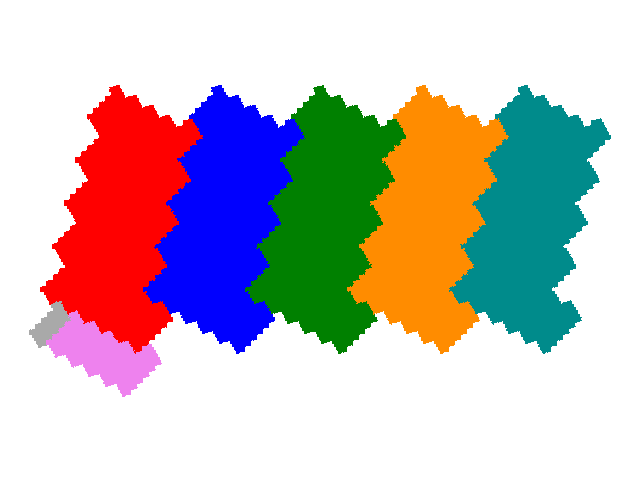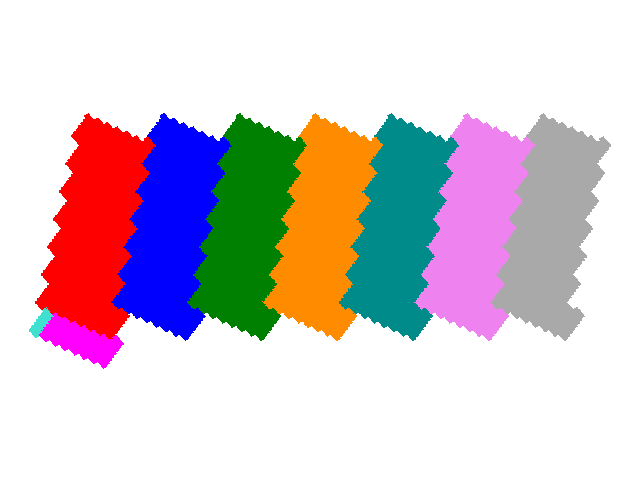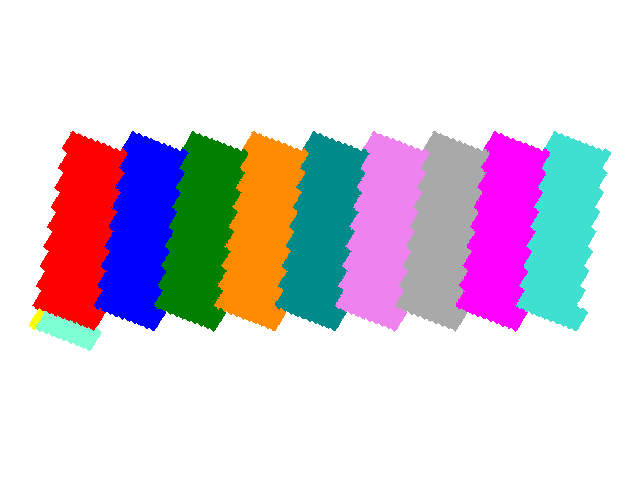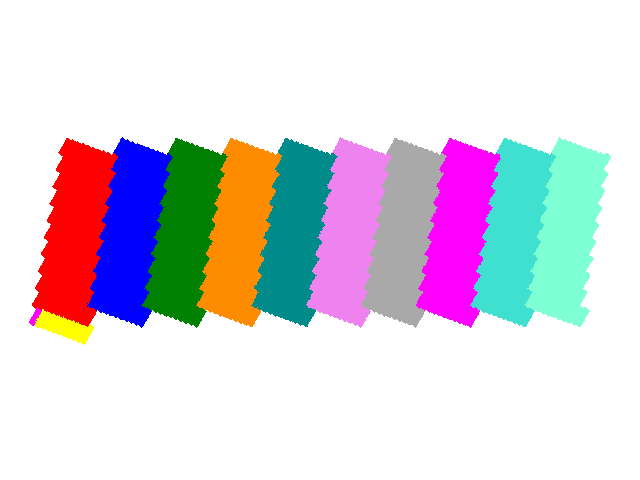All of these figures tile the plane with one copy per unit cell. The tiling vectors are a-1 and n + a.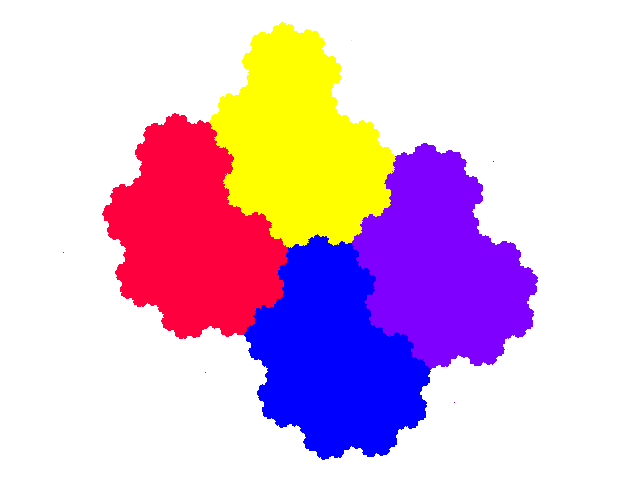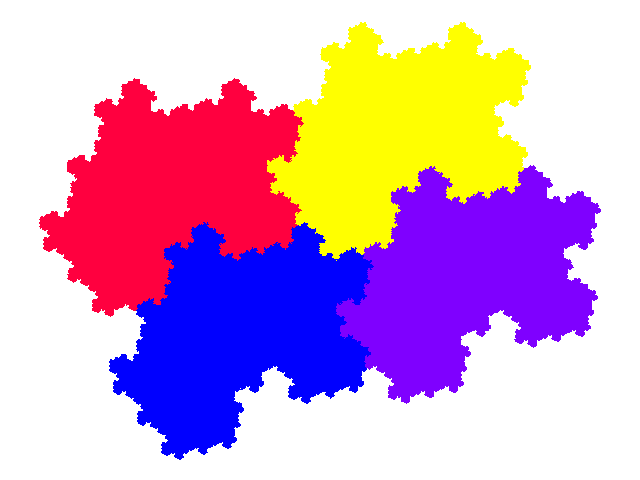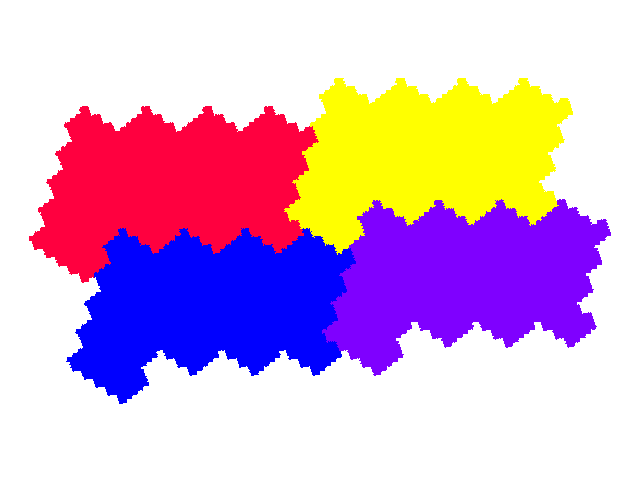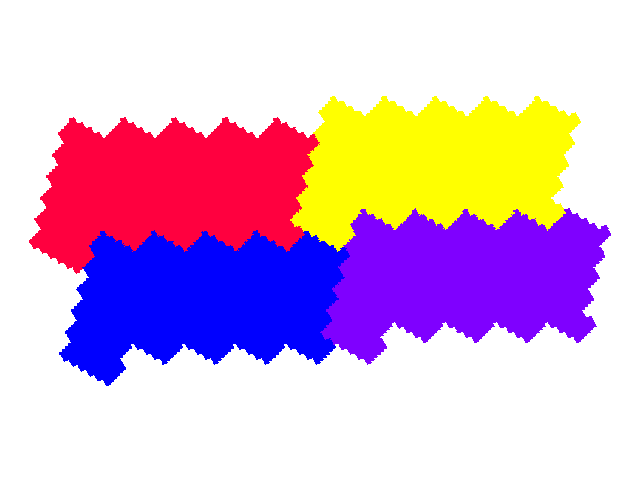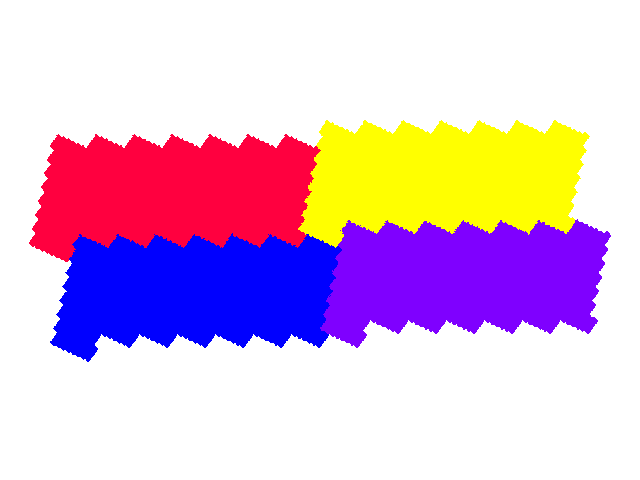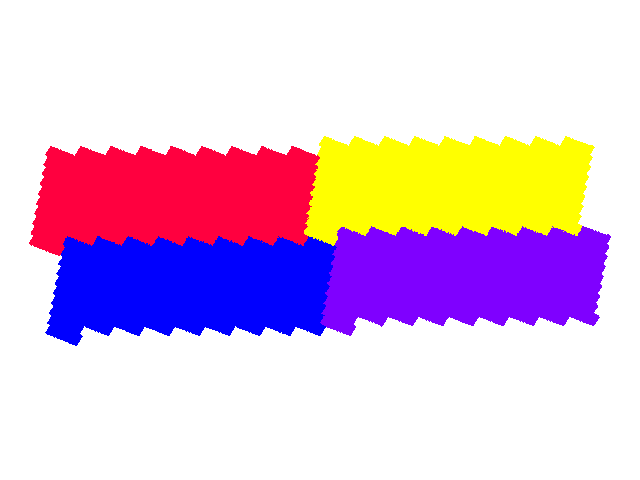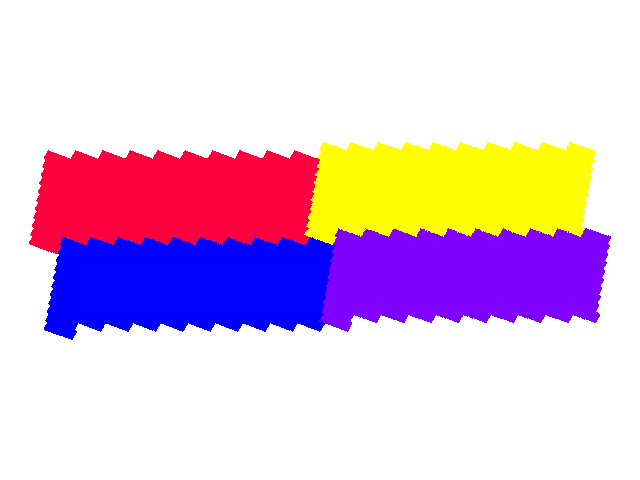Additional tiles can be generated by modification of the corresponding IFSs; I document some elsewhere for the 8th (n = 2) and 12th (n = 3) unit cubic Pisots.

Source: Reconstruction (n=2) of a tile published by Richard Kenyon; for n=3 and above these tiles are my own discoveries, from 2002. Tilings added in 2016.

References

© 2002, 2005, 2016 Stewart R. Hinsley